ISEE Middle Level Math : How to find percentage

Example Questions

Example Question #121 : Percentage

What is the decimal equivalent of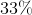?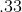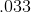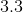Explanation:

Divide by 100: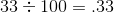Answer:Example Question #122 : Percentage

What is the decimal equivalent of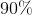?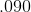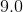Explanation:

Divide by 100: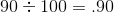Answer: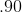Example Question #123 : Percentage

What is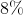written as a decimal?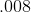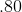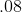Explanation:

Divide by 100: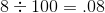Answer:Example Question #124 : Percentage

What is the decimal equivalent of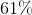?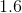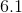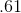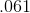Explanation:

Divide by 100: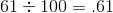Answer:Example Question #125 : Percentage

What is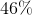written as a decimal?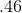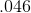Explanation:

Divide by 100: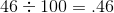Answer:Example Question #126 : Percentage

What is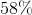in decimal form?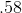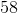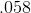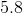Explanation:

Divide the percentage value by 100 to get the decimal equivalent: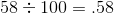Answer:Example Question #127 : Percentage

What is the decimal equivalent to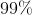?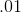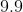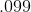Explanation:

Divide the percentage value by 100 to get the decimal equivalent: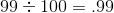Answer: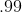Example Question #128 : Percentage

Which answer choice is equivalent to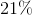?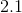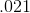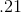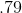Explanation:

Divide the percentage value by 100 to get the decimal equivalent: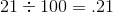Answer:Example Question #129 : Percentage

Write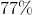as a decimal.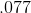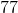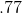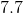Explanation:

Divide the percentage value by 100 to get the decimal equivalent: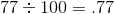Answer:Example Question #130 : Percentage

What is the decimal equivalent to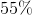?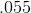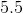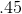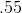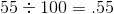Answer: